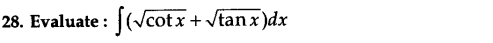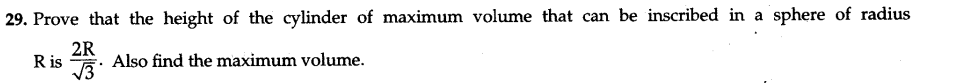# CBSE Sample Papers for Class 12 Maths 2016 Set 1

0
1007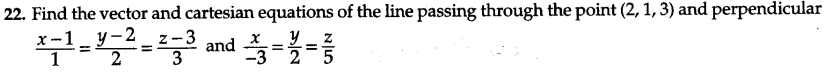SECTION — A

Question numbers 1 to 6 carry 1 mark each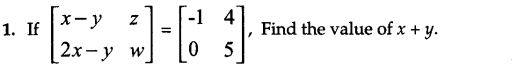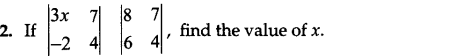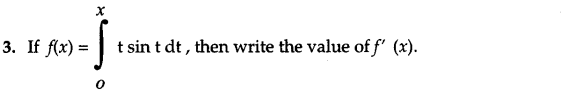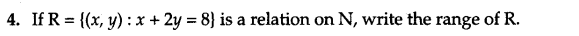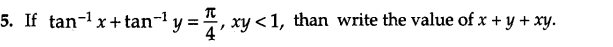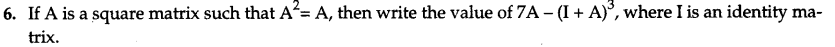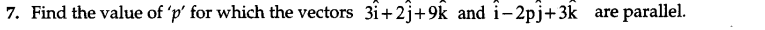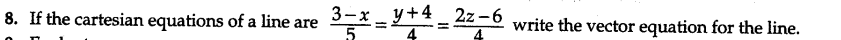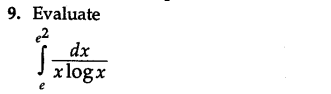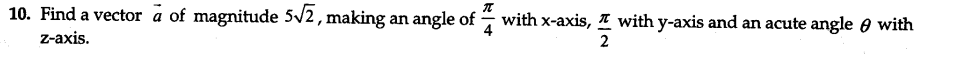SECTION — B

Question numbers 11 to 22 carry 4 marks each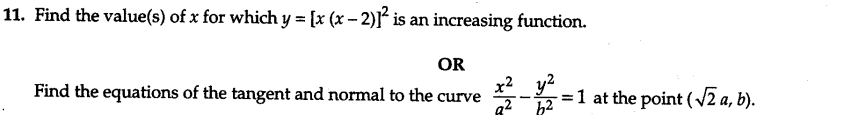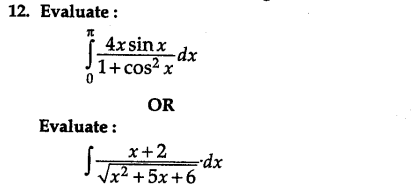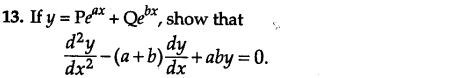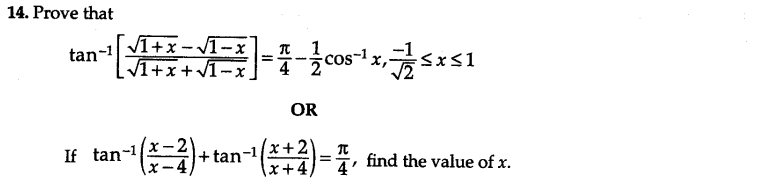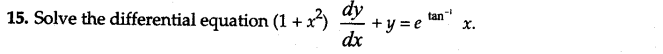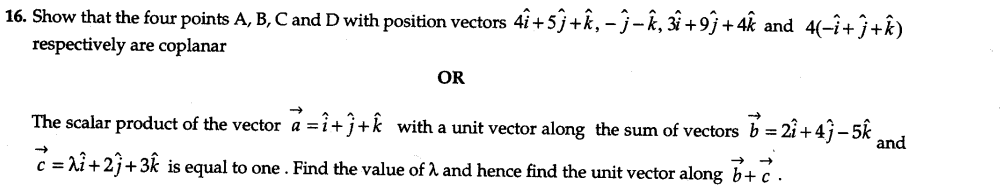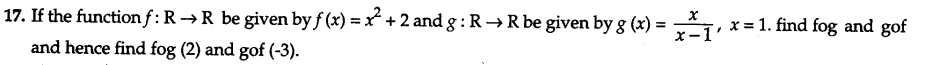18.An experiment succeeds thrice as often as it fails. Find the probability that in the next five trials, there will be at least 3 successes.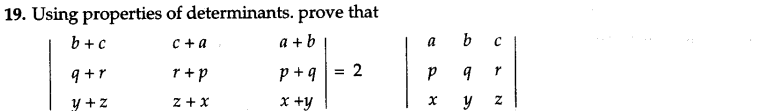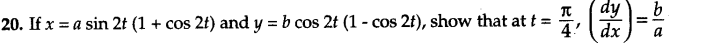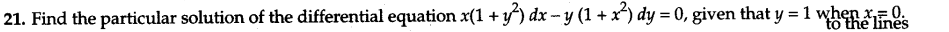SECTION — C

Question numbers 23 to 29 carry 6 marks each.
23.A manufacturing company makes two types of teaching aids A and B of Mathematics for class XII. Each type of A requires 9 labour hours of fabricating and 1 labour hour for finishing. Each type of B re­quires 12 labour hours for fabricating and 3 labour hours for finishing. For fabricating and finishing, the maximum labour hours available per week are 180 and 30 respectively. The company makes a profit of Rs 80 on each piece of type A and Rs 120 on each piece of type B. How many pieces of type A and type B should be manufac­tured per week to get a maximum profit ? Make it as an LPP and solve graphically. What is the maximum profit per week ?

24.There are three coins. One is a two-headed coin (having head on both faces), another is a biased coin that comes up heads 75% of the times and third is also a biased coin that comes up tails 40% of the times. One of the three coins is chosen at random and tossed, and it shows heads. What is the probability that it was the two-headed coin ?
OR
Two numbers are selected at random (without replacement) from the first six positive integers. Let X denote the larger of the two numbers obtained. Find the probability distribution of the random variable X, and hence find the mean of the distribution.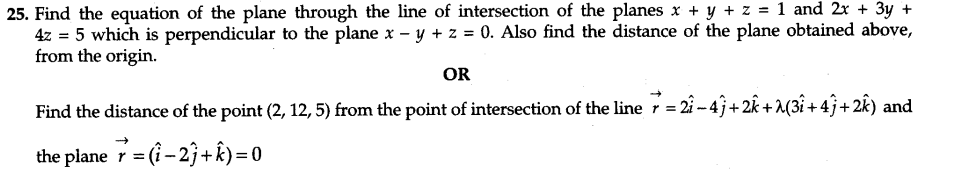26.Two schools A and B want to award their selected students on the values of sincerity, truthfulness and helpfulness. The school A wants to award Rs x each, Rs y each and Rs z each for the three respective values to 3,2 and 1 students respectively with a total award money of Rs 1,600. School B wants to spend Rs 2,300 to award its 4,1 and 3 students on the respective values (by giving the same award money to the three values as before). If the total amount of award for one prize on each value is Rs 900, using matrices, find the award money for each value. Apart from these three values, suggest one more value which should be considered for award.

27.Using integration, find the area of the region bounded by the triangle whose vertices are (-1,2), (1,5) and (3,4).